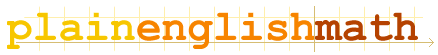You are here: MathematicsCalculus → Calculus

Calculus

Calculus concerns two topics: Integration and Differentiation. Each is the opposite of the other.

If you want to know what these two words actually mean, you'll have to read on...

Integration and Differentiation
The briefest possible introduction to what calculus is all about.

coming soon...

How To Differentiate Functions

How To Integrate Functions